# Tensor bundle

(diff) ← Older revision | Latest revision (diff) | Newer revision → (diff)

of type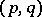on a differentiable manifoldThe vector bundle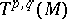overassociated with the bundle of tangent frames and having as standard fibre the space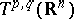of tensors (cf. Tensor on a vector space) of typeon, on which the groupacts by the tensor representation. For instance,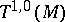coincides with the tangent bundleover, while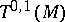coincides with the cotangent bundle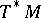. In the general case, the tensor bundle is isomorphic to the tensor product of the tangent and cotangent bundles:Sections of the tensor bundle of typeare called tensor fields of typeand are the basic object of study in differential geometry. For example, a Riemannian structure onis a smooth section of the bundle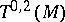the values of which are positive-definite symmetric forms. The smooth sections of the bundleform a module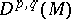over the algebra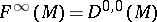of smooth functions on. Ifis a paracompact Hausdorff manifold, then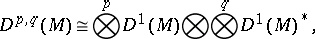where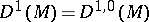is the module of smooth vector fields,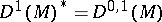is the module of Pfaffian differential forms (cf. also Pfaffian form), and the tensor products are taken over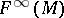. In classical differential geometry tensor fields are sometimes simply called tensors on.

How to Cite This Entry:
Tensor bundle. Encyclopedia of Mathematics. URL: http://encyclopediaofmath.org/index.php?title=Tensor_bundle&oldid=17758
This article was adapted from an original article by A.L. Onishchik (originator), which appeared in Encyclopedia of Mathematics - ISBN 1402006098. See original article